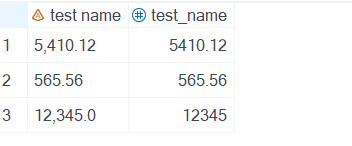## sas import excel give issue to convert numeric value

Hello,

I do have excel file which contains number in string format. Column header name is with space and I want to import file in SAS and convert string to numeric by using input function or something similar. SAS variable name should be sas naming format (space remove by _).

 test name 5,410.12 565.56 12,345.00

``````proc import out=test
datafile='C:\desktop\test.xlsx'
dbms=xlsx replace;
getnames=yes;
run;

data test;
set test;
test_name = input('test name'n, best12.);
run;``````

I need to create something like following output:5 REPLIES 5

## Re: sas import excel give issue to convert numeric value

If the cells in the column in the Excel worksheet only contain numbers (whatever style is used to display them) then SAS will make a numeric variable from the column.  Otherwise SAS will be  forced to make a character variable so that it can hold the character cell values. Any cell that was a number will be converted to a digit string that represents the number in the cell (note this include dates).

If you set the VALIDVARNAME=V7 option then SAS should automatically try to convert the column headers into valid SAS names.  It will only create variables with invalid names if you have (accidentally?) set the VALIDVARNAME option to ANY instead.

You can use the COMMA informat to convert strings that contain commas, dollar signs and percent signs into numbers.  The INPUT() function does not care if width used on the informat specification is larger than the length of the string being read.  32 is the maximum width that the COMMA informat can handle.

``````data test_fixed;
set test;
test_name = input('test name'n, comma32.);
run;``````

PS  BEST is the name of FORMAT, not an INFORMAT.  If you use it as an informat SAS will silently switch to using the normal numeric informat.  Just like if you accidentally use DOLLAR as the name of an informat it will switch to using the COMMA informat.

## Re: sas import excel give issue to convert numeric value

``````option validvarname=v7;
proc import out=test
datafile='C:\desktop\test.xlsx'
dbms=xlsx replace;
getnames=yes;
run;

proc print data=test (obs=5) noobs;
run;``````

What does that show?

## Re: sas import excel give issue to convert numeric value

Still comma became period when I tried to import the file.

## Re: sas import excel give issue to convert numeric value

@dht115 wrote:

Still comma became period when I tried to import the file.

What does that mean?

Are you in some location where the convention is to use comma as the decimal point and period as the thousands group separators?

## Re: sas import excel give issue to convert numeric value

```proc import out=test
datafile='C:\data\test.xlsx'
dbms=xlsx replace;
getnames=yes;
run;

data test (drop = test_name_import);
set test (rename =(Test_name = test_name_import));
format test_name best12.;
test_name = input(test_name_import, comma32.);
run;```
Discussion stats
• 5 replies
• 255 views
• 0 likes
• 4 in conversation